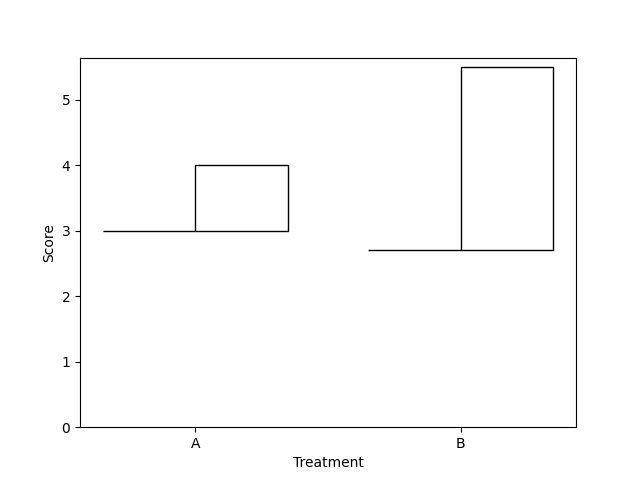# hat graphs need an example…

New plot format.

It would be really cool if matplotlib also support hat graphs.

They are a small difference from `bar` plots by removing the baseline part of the bar’s.
See here for details:
https://cognitiveresearchjournal.springeropen.com/articles/10.1186/s41235-019-0182-3Full dicussion on github.com

## 1 possible answer(s) on “hat graphs need an example…”

1. I didn’t read the whole paper, but if all it is is starting the bars at some other baseline, then this can be achieved using the `bottom` keyword argument:

```import matplotlib.pyplot as plt
import numpy as np

labels = ['A', 'B']
result1 = np.array([3, 2.7])
result2 = np.array([4, 5.5])

x = np.arange(len(labels))
width = 0.35

fig, ax = plt.subplots()
rects1 = ax.bar(x - width/2, np.zeros_like(result1), width,
bottom=result1, fill=False)
rects2 = ax.bar(x + width/2, result2 - result1, width,
bottom=result1, fill=False)

ax.set_xticks(x)
ax.set_xticklabels(labels)
ax.set_xlabel('Treatment')
ax.set_ylim(bottom=0)
ax.set_ylabel('Score')

plt.show()```